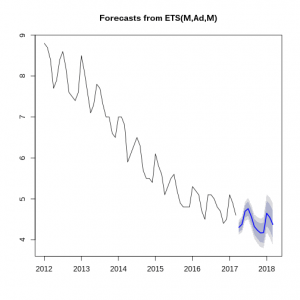Want to share your content on R-bloggers? click here if you have a blog, or here if you don't.Exponential smoothing is a method of finding patterns in time series, which can be used to make forecasts. In its simple form, exponential smoothing is a weighted moving average: each smoothed value is a weighted average of all past time series values (with weights decreasing exponentially from the most recent to the oldest values). In more complicated forms, exponential smoothing is applied to a time series recursively to allow for a trend and seasonality. In that case, the model is said to consist of three components – error, trend, and seasonality, from which another notation for exponential smoothing (“ETS”) is derived.
This set of exercises focuses primarily on the `ets` function from the `forecast` package. The function can be used to apply various exponential smoothing methods (including Holt’s and Holt-Winters’ methods), and allows for both automatic and manual selection of the model structure (for example, whether the model includes trend and seasonal components). The exercises are based on the monthly data on US civilian unemployment rate as a percentage of the labor force for 2012-2017 retrieved from FRED, the Federal Reserve Bank of St. Louis database (download here)

For other parts of the series follow the tag forecasting.
Answers to the exercises are available here.

Exercise 1
Load the data, transform it the the `ts` type (indicating that the data is monthly and the first period is January 2012), and plot it.

Exercise 2
Use the `ses` function from the `forecast` package to get a forecast based on simple exponential smoothing for the next 12 months, and plot the forecast.

Exercise 3
Estimate an exponential smoothing model using the `ets` function with default parameters. Then pass the model as input to the `forecast` function to get a forecast for the next 12 months, and plot the forecast (both functions are from the `forecast` package).

Exercise 4
Print a summary of the model estimated in the previous exercise, and find the automatically estimated structure of the model. Does it include trend and seasonal components? If those components are present are they additive or multiplicative?

Exercise 5
Use the `ets` function to estimate an exponential smoothing model with a damped trend. Make a forecast based on the model for the next 12 months, and plot it.

Learn more about Forecasting in the online course Time Series Analysis and Forecasting in R. In this course you will learn how to:

• A complete introduction on Forecasting
• Work thru an exponentional smoothing instruction
• And much more

Exercise 6
Use the `ets` function to estimate another model that does not include a trend component. Make a forecast based on the model for the next 12 months, and plot it.

Exercise 7
Find a function in the `forecast` package that estimates the BATS model (exponential smoothing state space model with Box-Cox transformation, ARMA errors, trend and seasonal components). Use it to estimate the model with a dumped trend, and make a forecast. Plot the forecast.

Exercise 8
Use the `accuracy` function from the `forecast` package to get a matrix of accuracy measures for the forecast obtained in the previous exercise. Explore the structure of the matrix, and save a measure of the mean absolute error (MAE) in a variable.

Exercise 9
Write a function that inputs a time series and a list of model estimation functions, calculates forecasts for the next 12 periods using each of the functions (with default parameters), and outputs the forecast with the smallest mean absolute error.
Run the function using the `unemployment` time series and a list of functions that includes `ets`, `bats`, and `auto.arima`. Plot the obtained result.

Exercise 10
Modify the function written in the previous exercise so that it prints the mean absolute error for each forecasting model along with the name of that model (the name can be retrieved from the `forecast` object).